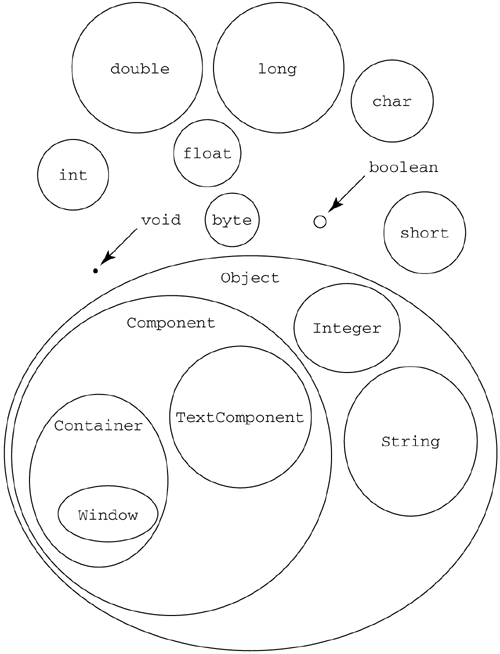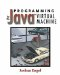# 10.2 Expression Types

The Java type system is slightly different from the one in the JVM, but they are very similar, and the JVM type system is used to implement the Java type system.

Java has eight numeric types: int, double, long, float, byte, boolean, short, and char. These types designate entities from a fixed universe. These entities do not have to be created; they just exist.

The other kind of type is the object type. Classes are the types of objects. Each time an object is created, a new element is added to the set of elements of that class. The object is said to be an instance of that class. All of the instances of the class are in the set of that class.

Each class has a superclass, except for the special class java.lang.Object. All instances of that class are also instances of the superclass. The instances of the subclass form a subset of the instances of the superclass. Another way of saying this is that the subclass is a subtype of the superclass.

The term subtype refers to the type and all of the types within it. A type is always a subtype of itself. All the subtypes of a type, but not the type itself, are called the proper subtypes of that type.

Figure 10.2 shows a diagram of part of the Java type system. To make it fit more easily onto a page, package names have been omitted. All the classes depicted are part of the standard Java platform. Many others have been omitted.

##### Figure 10.2. Part of the Java type system#### 10.2.1 Numeric Types

At the top of Figure 10.2 are the numeric types: int, long, and so forth. The relative sizes give a rough idea of how many values are in that type. The figure is not drawn to scale: long is actually 4 billion times larger than int. The type boolean contains just two members: true and false. The special type void actually contains no members. It is used as a placeholder type for methods that don't return a value.

Notice that all the numeric types are disjoint. The ints are not a subset of the longs, and the shorts, bytes, and chars are not a subset of the ints. This is different from the JVM itself, where shorts, ints, bytes, chars, and booleans are all represented the same way.

To convert from an int value 1 to a short value, a special conversion operation must be performed, which maps the elements from the set of ints onto the elements of the set of shorts. This operation is called a coercion. Sometimes the Java compiler performs the coercion automatically (see section 1.8.1), but it always happens when you want to use a value of one type as a value of another.

Similar conversions may be performed on almost any pair of numeric types. However, you can't convert a boolean to or from anything else. Since there are no elements in the set of void, no conversions are possible there, either.

#### 10.2.2 Object Types

At the bottom of Figure 10.2 is the set of classes, with subclasses shown as smaller bubbles within other bubbles. Each bubble is entirely contained within exactly one other (except for Object, which occupies a privileged position at the top). The figure shows that a Window is a Container, which is a Component, which is an Object. A String is also an Object, but a String is not a Component, nor is a Component a String.

An interface is like a class, except that it may span multiple supertypes. All members of any class that implements the interface are included in the domain of the interface. From the point of view of an expression, an interface type is treated identically to a class type, except when it is used as the receiver of a method invocation.

#### 10.2.3 Expression Result Types

When an expression is executed, it yields a result. Executing an expression is sometimes called evaluating the expression. Each result is an entity from somewhere in the type system; each result has a type. The type of the result actually produced when the expression is executed is called the runtime type.

The runtime type of an expression is not always the same each time an expression is executed. For example, consider this expression:

` vector.elementAt(5) `

where vector is an object of type java.util.Vector. The return type of the elementAt method in Vector is Object. This means that whatever is returned will fit somewhere in the domain of Object. The exact runtime type may be a String or a Window or any other kind of object, depending on what the program put into the vector.

It is impossible for a Java compiler to predict the exact runtime type of an expression. It may even be different each time the expression is evaluated. However, it is always possible for the compiler to deduce something about the runtime type. For example, in this case the compiler can deduce from the declared return type of the elementAt method that the returned value will be some sort of Object. This doesn't tell you a lot about the result, but it does tell you that it isn't an int or a long or other numeric type.

The most specific thing the compiler can deduce about the type is called the compile-time type of the expression. The compiler uses rules like these to deduce the compile-time type of each expression:

• The compile-time type of adding two ints together is another int.

• The compile-time type of invoking a method is the return type of that method.

• The compile-time type of a constant value is the type of that constant.

• The compile-time type of reading a field is the type of that field.

• The compile-time type of reading a local variable is the declared type of that local variable.

And so on.

The rules guarantee that the runtime type of the result will either be the same as the compile-time type of the expression or some subtype of the compile-time type. The runtime type is said to conform to the compile-time type.

Since the numeric types don't have subtypes in Java, the runtime type of an expression that returns a numeric type is always exactly the same as the compile-time type. If the type is an object type, then the runtime type may be a subtype of the compile-time type.

#### 10.2.4 Compile-Time Types and Type Restrictions

Types restrict what operations can be performed on values of that type. The compiler uses the compile-time type to deduce what sorts of operations will be legal on the runtime type. For example, look at these declarations:

` class Point {    // Definition omitted } class Rectangle {    boolean contains(Point p)   {/* implementation omitted */ }    void move(Point p)          {/* implementation omitted */ } } int a,b; float f1, f2; Point p; Rectangle r; Object o; `

##### Table 10.2. Examples of compile-time types
Expression Compile-time type
a + b int
f1 * f2 float
r.contains(p) boolean
o.toString() java.lang.String
r.toString() java.lang.String
o = r java.lang.Object
r.move(p) void

Some legal expressions are given in Table 10.2. The last one doesn't actually produce a value, since there are no members of the type void. The type void is used to make sure that every expression has a type. Since no operations apply to void values, the result of this expression can't be used as part of other expressions.

Illegal expressions are given in Table 10.3. In the last one, it may be that o is really a Rectangle, since Rectangles are included in the domain of Objects. If this were the case, then the method call to contains would be legal, since there is a method called contains in Rectangle that takes a Point as its argument. However, the Java compiler can work only on the basis of what it can prove when it is compiling. Since the object in o might not be a Rectangle, the Java compiler flags the expression o.contains(p) as illegal.

#### 10.2.5 Type Safety and Verifiability

The type rules discussed briefly here define which operations are legal and which are not. If a program does only legal things, then it is said to be type-safe. A Java program must be type-safe in order for the resulting bytecodes to be verifiable by the bytecode verification algorithm. If it is not type-safe, then the compiler will reject the program.

The reason that type-safe Java programs produce verifiable JVM bytecodes is that the type checking applied to JVM instructions is similar to the Java type deduction rules. The compile-time types used by the Java compiler to determine whether or not a program is safe is analogous to the rules applied by the JVM verification algorithm when verifying a program.

The key is that each expression produces a known compile-time type, and the code that is generated can be shown to always produce a value at runtime that conforms to that type. The various operations combine values of these types, producing results that are predictable, at least where type is concerned.

In forthcoming sections, as we discuss how various Java constructs translate into JVM code, you should check how the various constructs compile into code that produces values of a type that can be checked by the verification algorithm.

The discussion of Java types given here is extremely abbreviated. A full explanation of type safety can be found in chapter 13 of The Java Language Specification.

##### Table 10.3. Illegal expressions
Expression Illegal because...
p1 + p2 Points can't be added.
r * r Rectangles can't be multiplied.
r = o The object identified by o may not be a Rectangle.
r = 5 An int is not a Rectangle.
a.move(p1, p2) There is no method called move in Rectangle that can take two Points.
r.contains(f1) There is no method called contains in Rectangle that can take a float.
r.contains(p, p) There is no method named contains in Rectangle that can take two Points.
o.contains(p) There is no method in Object named contains that takes a Point.Programming for the Javaв„ў Virtual Machine
ISBN: 0201309726
EAN: 2147483647
Year: 1998
Pages: 158
Authors: Joshua Engel

Similar book on Amazon